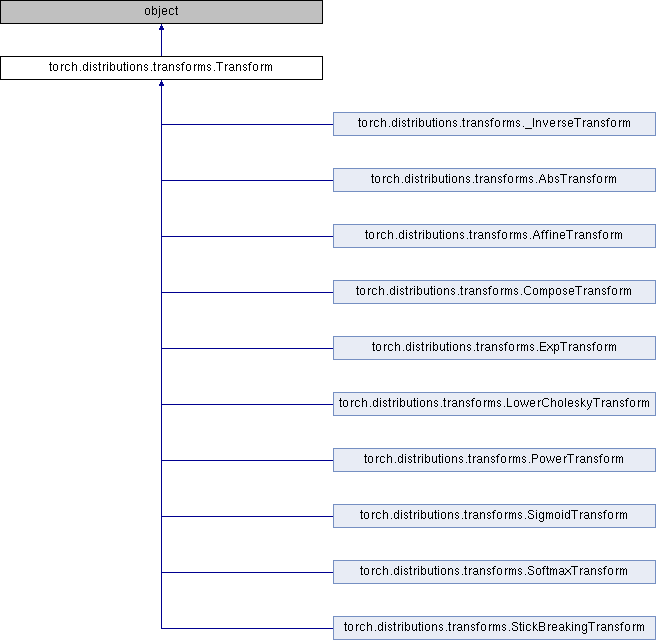Caffe2 - Python API A deep learning, cross platform ML framework
torch.distributions.transforms.Transform Class Reference
Inheritance diagram for torch.distributions.transforms.Transform:## Public Member Functions

def __init__ (self, cache_size=0)

def inv (self)

def sign (self)

def __eq__ (self, other)

def __ne__ (self, other)

def __call__ (self, x)

def log_abs_det_jacobian (self, x, y)

def __repr__ (self)

bijective

event_dim

## Detailed Description

```Abstract class for invertable transformations with computable log
det jacobians. They are primarily used in
:class:`torch.distributions.TransformedDistribution`.

Caching is useful for tranforms whose inverses are either expensive or
numerically unstable. Note that care must be taken with memoized values
since the autograd graph may be reversed. For example while the following
works with or without caching::

y = t(x)

However the following will error when caching due to dependency reversal::

y = t(x)
z = t.inv(y)
grad(z.sum(), [y])  # error because z is x

Derived classes should implement one or both of :meth:`_call` or
:meth:`_inverse`. Derived classes that set `bijective=True` should also
implement :meth:`log_abs_det_jacobian`.

Args:
cache_size (int): Size of cache. If zero, no caching is done. If one,
the latest single value is cached. Only 0 and 1 are supported.

Attributes:
domain (:class:`~torch.distributions.constraints.Constraint`):
The constraint representing valid inputs to this transform.
codomain (:class:`~torch.distributions.constraints.Constraint`):
The constraint representing valid outputs to this transform
which are inputs to the inverse transform.
bijective (bool): Whether this transform is bijective. A transform
``t`` is bijective iff ``t.inv(t(x)) == x`` and
``t(t.inv(y)) == y`` for every ``x`` in the domain and ``y`` in
the codomain. Transforms that are not bijective should at least
maintain the weaker pseudoinverse properties
``t(t.inv(t(x)) == t(x)`` and ``t.inv(t(t.inv(y))) == t.inv(y)``.
sign (int or Tensor): For bijective univariate transforms, this
should be +1 or -1 depending on whether transform is monotone
increasing or decreasing.
event_dim (int): Number of dimensions that are correlated together in
the transform ``event_shape``. This should be 0 for pointwise
transforms, 1 for transforms that act jointly on vectors, 2 for
transforms that act jointly on matrices, etc.
```

Definition at line 26 of file transforms.py.

## Member Function Documentation

 def torch.distributions.transforms.Transform.__call__ ( self, x )
```Computes the transform `x => y`.
```

Definition at line 117 of file transforms.py.

 def torch.distributions.transforms.Transform.inv ( self )
```Returns the inverse :class:`Transform` of this transform.
This should satisfy ``t.inv.inv is t``.
```

Definition at line 89 of file transforms.py.

 def torch.distributions.transforms.Transform.log_abs_det_jacobian ( self, x, y )
```Computes the log det jacobian `log |dy/dx|` given input and output.
```

Definition at line 155 of file transforms.py.

 def torch.distributions.transforms.Transform.sign ( self )
```Returns the sign of the determinant of the Jacobian, if applicable.
In general this only makes sense for bijective transforms.
```

Definition at line 103 of file transforms.py.

The documentation for this class was generated from the following file: